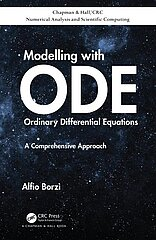# New book available

03/04/2020

Alfio Borzì: Modelling with Ordinary Differential Equations: A Comprehensive Approach

### Summary

Modelling with Ordinary Differential Equations: A Comprehensive Approach aims to provide a broad and self-contained introduction to the mathematical tools necessary to investigate and apply ODE models. The book starts by establishing the existence of solutions in various settings and analysing their stability properties. The next step is to illustrate modelling issues arising in the calculus of variation and optimal control theory that are of interest in many applications. This discussion is continued with an introduction to inverse problems governed by ODE models and to differential games.

The book is completed with an illustration of stochastic differential equations and the development of neural networks to solve ODE systems. Many numerical methods are presented to solve the classes of problems discussed in this book.

Features:

• Provides insight into rigorous mathematical issues concerning various topics, while discussing many different models of interest in different disciplines (biology, chemistry, economics, medicine, physics, social sciences, etc.)
• Suitable for undergraduate and graduate students and as an introduction for researchers in engineering and the sciences
• Accompanied by codes which allow the reader to apply the numerical methods discussed in this book in those cases where analytical solutions are not available